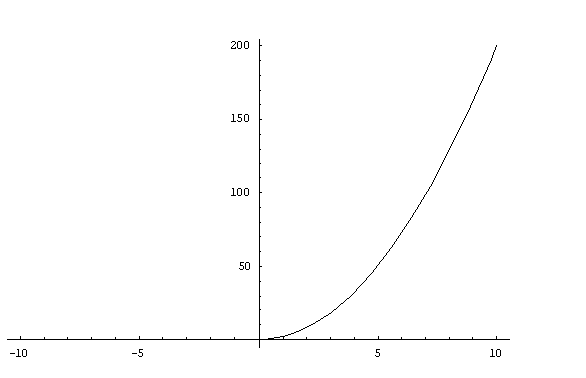#### You may also like### How Many Solutions?

Find all the solutions to the this equation.### Quartics

Investigate the graphs of y = [1 + (x - t)^2][1 + (x + t^)2] as the parameter t varies.### Power Up

Show without recourse to any calculating aid that 7^{1/2} + 7^{1/3} + 7^{1/4} < 7 and 4^{1/2} + 4^{1/3} + 4^{1/4} > 4 . Sketch the graph of f(x) = x^{1/2} + x^{1/3} + x^{1/4} -x

# Slide

##### Age 16 to 18 Challenge Level:

This solution was written by Andrei from Tudor Vianu National College, Bucharest, Romania.

I calculate the values of $f(x)= x(x+|x|)$ for $x< 0$ and $x\geq 0$:

\eqalign{ f(x) &= x(x-x)=0\ for \ x < 0 \cr &=x(x+x)= 2x^2 \ for \ x\geq 0.}

Its graph is represented below:Now, I find the first derivative of $f(x)$:

\eqalign{ f'(x) &= 0\ {\rm for}\ x < 0 \cr &= 4x \ {\rm for}\ x\geq 0}

I observe that for $x = 0$, $f'(x)$ is $0$ both from the first and from the second form of $f(x)$, that is on both sides of the origin. So the first derivative $f'(0)=0$ exists at $x=0$. Hence the graph of $f(x)$ has the tangent at $y=0$ at the origin.

Now, I calculate the second derivative:

\eqalign{ f''(x) &= 0\ for\ x < 0 \cr &= 4\ for \ x> 0.}

Hence the second derivative does not exist at the origin because on the left the limiting value of $f''(x)$ as $x\to 0$ is $0$ whereas on the right the limiting value of $f''(x)$ as $x\to 0$ is $4$. So there isn't a unique tangent to the graph of $f'(x)$ at $x = 0$.